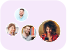Product Edition:2nd Edition
Author: Kunze Hoffman
Book Name: Linear Algebra
Subject Name: Maths

# Linear Algebra 2nd Edition Solutions

0 out of 5.0
29 reviews15 Students
have requested for homework help from this book

Principles Of Mathematical Analysis is the first in Walter Rudinâ€™s series of books on mathematical analysis and is a leading introductory text on the subject. Summary of the Book Principles of Mathematical Analysis provides a comprehensive introduction to some of the major theories of mathematical analysis. First published in 1953, the book is a standard text for undergraduate and first year graduate students. The book begins with a discussion on Real and Complex numbers and Basic Topology. Here, students are introduced to the background required to understand rest of the topics in the book. The book goes on to discuss Numerical Sequences and Series, Continuity, Differentiation, Riemann-Stieltjes Integral, Integration of Differential Forms, and The Lebesgue Theory. Appropriate appendices and exercises follow each chapter. The book, in its third edition, includes a new section on the gamma function, and interesting, new exercises.

5
15
4
6
3
2
2
1
1
5

0

## Students who viewed this book also checked out